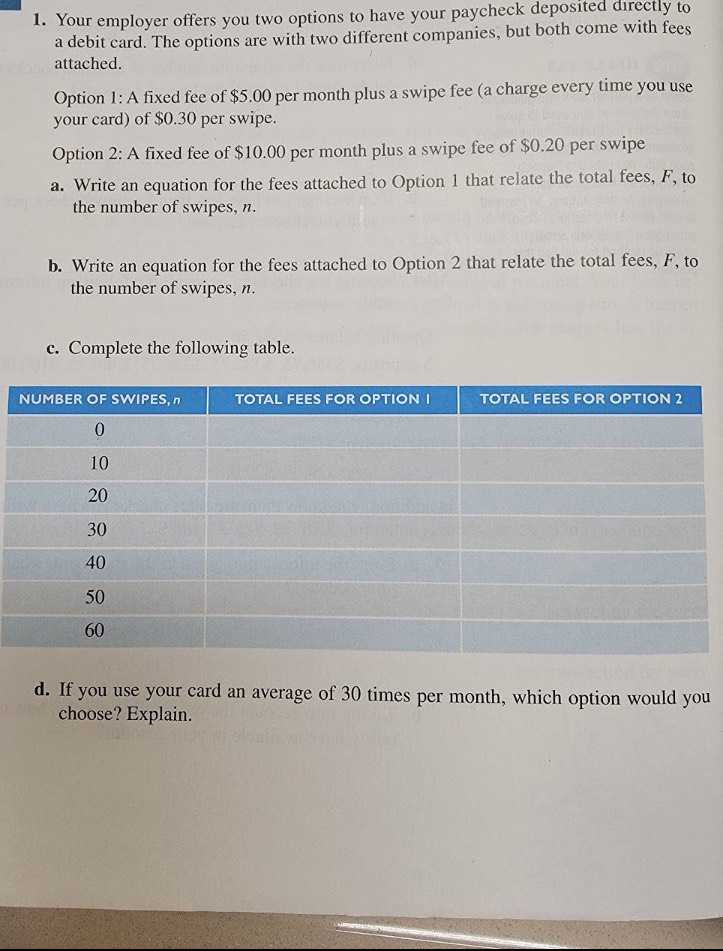### ¿Todavía tienes preguntas de matemáticas?

Pregunte a nuestros tutores expertos
Algebra
Pregunta1. Your employer offers you two options to have your paycheck deposited directly to a debit card. The options are with two different companies, but both come with fees attached.

Option 1: A fixed fee of $$\ 5.00$$ per month plus a swipe fee (a charge every use you use your card) of $$\ 0.30$$ per swipe.

Option 2: A fixed fee of $$\ 10.00$$ per month plus a swipe fee of $$\ 0.20$$ per swipe

a. Write an equation for the fees attached to Option $$1$$ that relate the total fees, $$F$$ , to the number of swipes, $$n$$ .

b. Write an equation for the fees attached to Option $$2$$ that relate the total fees, $$F$$ , to the number of swipes, $$n$$ .

c. Complete the following table.

d. If you use your card an average of $$30$$ times per month, which option would you choose? Explain.